﻿ Gravitational wave does NOT exist !

# Gravitational wave is fiction.

## Gravitational wave is very doubtful.

### [ Gravitational wave is too weak to believe, and useless. ]

(Fig.1) Gravitational wave changes the arm by only 1/1000 of a proton ?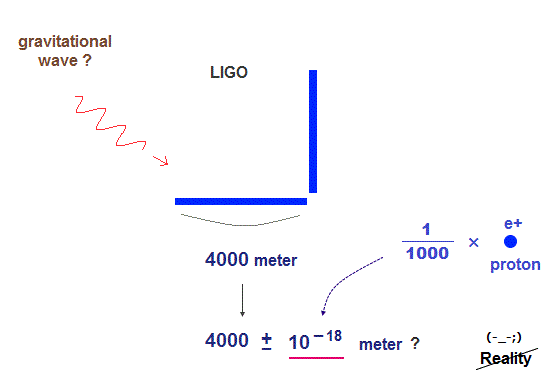There is a rumor that elusive gravitational wave may have been found in twitter.  Unfortunately, the gravitational wave doesn't exist.  See this.

Physicists should Not repeat the same kind of fraud as BICEP2.
They must honestly admit and reveal Einstein fatal flaws !

First, the gravitational wave is too weak to confirm, so very doubtful.

Gravitational wave changes the arm length of LIGO by only a thousandth of a proton (= 10-18 meter  change ) !  ← Too small.

So there are many other factors that cause this extremely small change.
This decision to admit fanciful gravitational wave is very "political", not science !

## Pulsar cannot prove gravitational wave.

### [ Only 76 microsecond change per year ← very doubtful ! ]

(Fig.2) The experimental proof using pulsar (?) is very doubtful.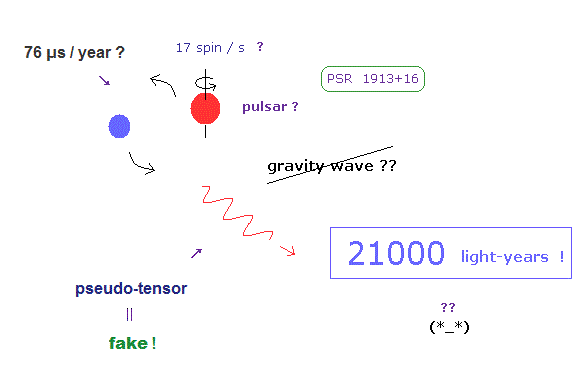It is said that this doubtful gravitation wave was indirectly confimed by observing the pulsar's light signal.

But in fact, this experimental result lacks credibility.
This binary pulsar is belived to be two neutron stars.

But the pulsar is too far (= 21000 light years ! ) away from the earth to see.
We can observe only light pulse change emitted from some unknown objects.

They argue the orbital period of this pulsar is 7.7 hours, and its period decreases slightly by 0.000076 seconds per a year by emitting gravitational wave.

This too faint change shows the experimental proof of gravitational wave cannot be trusted at all.

The light pulse emitted from objects which is 21000 light-year away from the earth is likely to be affected by various dusts on its long way.

## Gravitational wave is "fake" tensor.

### [ Einstein relativity cannot conserve energy → pseudotensor ? ]

(Fig.3) Gravitational pseudo-tensor contradicts Einstein relativity.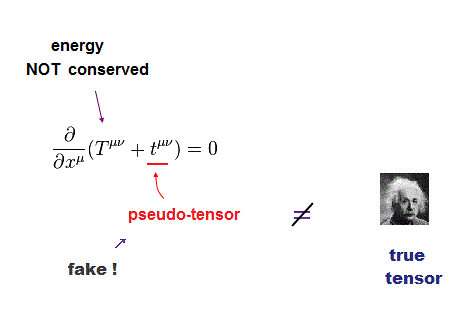Though the media likes to say gravitational wave is one of predictions (← ? ) of Einstein relativity, it's a big lie.

Gravitational wave is called "pseudo-tensor", which is not a true tensor of Einstein relativity.  This pseudotensor vanishes in some coordinate we choose.

This means the gravitational wave pseudotensor is NOT a real object.
Why did they have to introduce this absurd gravitational wave ?

In fact, Einstein general relativity cannot conserve energy ( this p.2 ) !
So they artificially added unrelated pseudo-tensor (= t ) to the original true tensor T.

Of course, this pseudo-tensor is not a true tensor, so many physicists including Einstein rejected this gravitational wave.

So the gravitational wave is NOT the Einstein's greatest prediction at all !

## Gravitational wave can vanish, so illusion.

### [ Gravitational wave vanishes in some "chosen" coordinate. ]

(Fig.4) ↓ Reason why gravitational wave is illusion.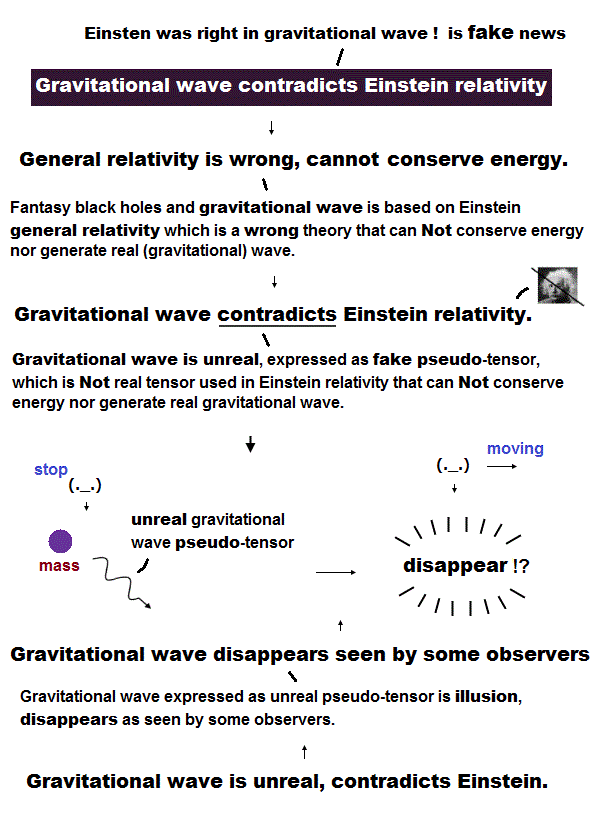If gravitational wave is "real wave" agreeing with Einstein relativity, it must keep its form independent of any coordinate ( ex. by observer's motion ).

But it cannot.  In fact, this gravitational wave vanishes in some chosen coordinate ( this p.2 ).

This means there is NO concept such as "energy conservation" in gravitational wave, so unreal.

## There are many pseudotensors, so useless.

### [ We can "choose" any pseudotensor as gravitational wave. ]

(Fig.5) Pseudotensor has many candidates, cannot predict anything.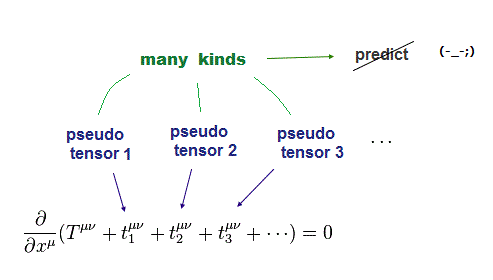There is No restriction in gravitational wave pseudotensor except ( fake ) energy conservation law.

So many different pseudotensors were invented by many physicists ( this p.2 ).  You just choose a convenient pseudotensor as gravitational wave out of many choices.

Of course, different pseudo-tensors give different energy values ( this p.3, this p.17 ).

It means gravitational wave pseudotensor has NO ability to predict energy values, so useless.  And the pulsar's experiment is meaningless.

## Pseudotensor's energy is NOT determined.

### [ Artificial coordinate is needed for some pseudotensors to be the same. ]

(Fig.6) We can freely change the energies of gravitational waves !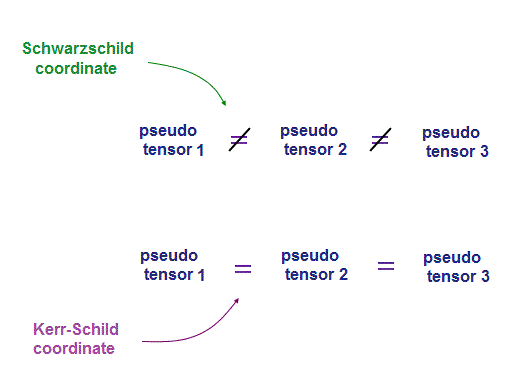In general relativity, "gravity" affects the spacetime structure.
So depending on the gravitational coordinate (= cartesian, spherical .. ) we choose, the results change.

The most accepted one is Schwarzschild spherical coordinate, as seen in black hole.  But this coordinate is incompatible with gravitational waves.

In most coordinates, different gravitational pseudotensors give different energy values.  Only under special coordinate, some of them agree with each other.

See this and this p.12.
This special one is called Kerr-Schild "Cartesian" coordinate ( this p.10 ).

Of course, if gravitational wave is real, its energy must be the same, independent of any chosen coordinate.

Choosing conveninent pseudotensor and coordinate is one of nonsense tricks in gravitational waves.

## Binary pulsar is unseen, just imaginary.

### [ The light pulse from pulsar ( ? ) is the only clue. ]

(Fig.7) They imagine pulsar motion only from the vague light pulse.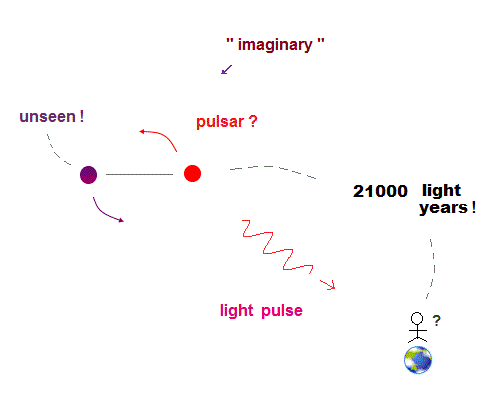It is said the binary pulsar's motion indirectly proved gravitational wave.
But these pulsars are so far away from the earth that we cannot see their motions !

They just imagine the motions of a pair of pulsars only from the light pulse.
They argue the light frequency or timing change give the pulsar's orbital period.

In fact, one of these pulsars cannot been seen (= not emit any light pulse ).
So we don't know this light frequency change really means two pulsars' orbital motions.

The problem is they cannot determine the masses of two pulsars only from usual Keplerian parameters.

They must estimate their masses using two vague parameters such as orbital precession and relativistic redshift ( this p.10 ).

Estimating all motional parameters ( this p.2 ) is very hard and speculative.  It's no wonder the light pulse ( this p.3 ) may change on its long way by other unrelated dusts or something.

## Gravitational wave effect is too weak to believe.

### [ Only 0.000075 seconds decrease per 1 year in orbital period ? ]

(Fig.8) Orbital period's change is too weak to confirm gravitational wave.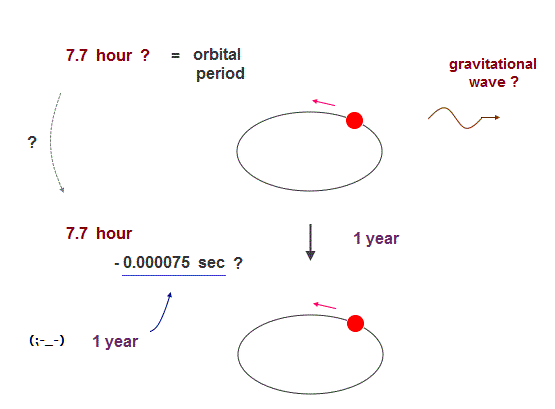They argue the orbital period of these pulsars is about 7.7 hours.
And it decreases by 0.000075 second per year by emitting gravitational wave.

This very slight change (= 75 μs / year ) in orbital period is too small to believe !

So it's very hard to say this observation proves the gravitational wave.
It's no wonder the light pulse changes by other factors on its long journey.

## Precession is much bigger than gravitational wave.

### [ Pulsar's precession makes gravitional wave's effect unclear. ]

(Fig.9) Pulsar precession is more than 1000 times bigger than wave.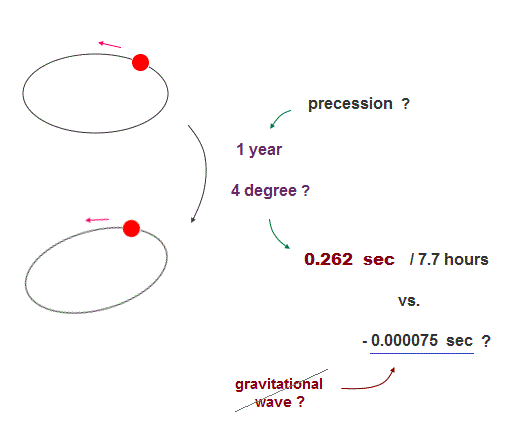The point is the orbit of these pulsar is NOT closed.
These orbits are precessing at 4 degrees per year, they argue.

Of course, we cannot see this pulsar's precession directly !
They just estimate it only from the slight change of the light pulse.

The problem is this precession's effect is more than 1000 times bigger than the weak gravitational wave.

To know the precise gravitational wave's effect, we have to define "one round of the closed orbit".  But this large precession makes it impossible.

This precession may affects the light frequency (= Doppler ) per revolution.  The pulsar is so distant that we cannot see their precise motions.

So very slight change in orbital period by gravitational wave is hidden in this large precession, and it's very hard to extract it.

So testing general relativity using this too faint gravitational wave is impossible.

## General relativity prediction is doubtful.

### [ Gravitational wave is too weak to test general relativity. ]

(Fig.10) ↓ This graph really proved gravitational wave ?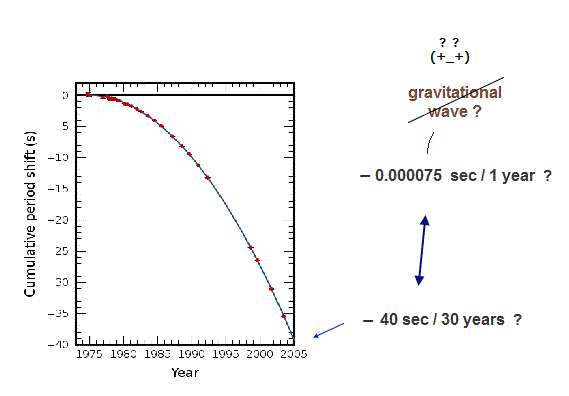You may often see this graph as general relativity prediction of elusive gravitational wave.  But in fact, this graph is very unreliable.

According to this graph, the "cumulative" period shift is as much as 40 second, which is much bigger than gravitational wave effect (= 75 microsecond / year ).

The reliability of this graph depends on how we know the precise orbital period without gravitational wave.

But it's impossible to distinguish very weak gravitational wave from the large precession, which prevents us from defining "one round of orbit".

Unless we define the precise circumference of the orbit (← not closed ! ), gravitational wave's effect is hidden in other effect.

## Energy-momentum tensor.

### [ Energy must be conserved in energy flux. ]

(Fig.11)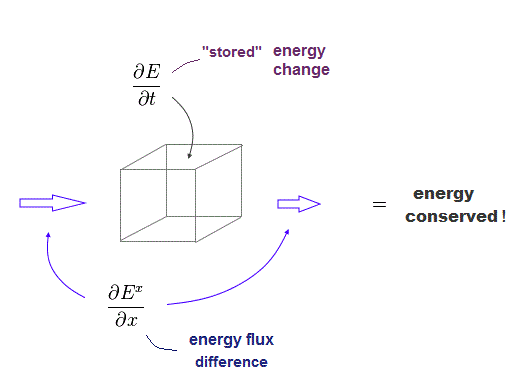Of course, total energy must be conserved.
The classical energy momentum tensors satisfy energy conservation.

Ex means the energy flux in x direction.
The difference in this energy flux is equal to energy change inside the space.

(Fig.12) Energy-momentum tensor satisfy energy conservation.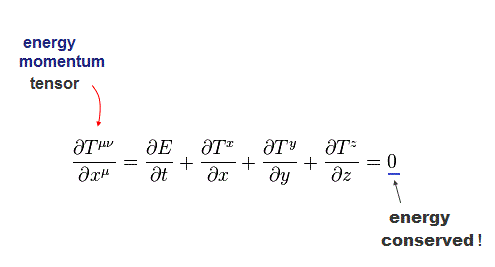The sum of energy flux in all directions is equal to the total energy change per time.  This is called "energy-momentum ( stress-energy ) tensor".

## Einstein tensor cannot conserve energy.

(Fig.13)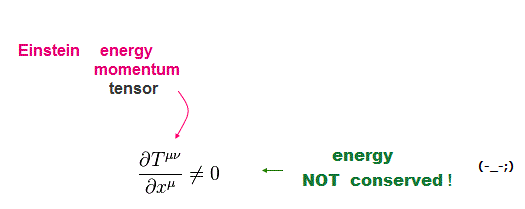The problem is that Einstein general relativity cannot conserve energy in his tensors.

(Fig.14)  ↓ Artificial pseudotensor is needed to conserve energy.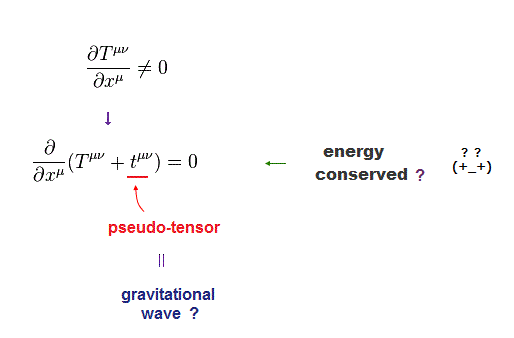So physicists add some artificial pseudotensor (= t ) to the original Einstein tensor (= T ) to meet energy conservation law by force.

This pseudotensor (= gravitational wave ) is NOT a true tensor.
Because Einstein true tensor does NOT conserve energy !

(Fig.15)  There are many different pseudotensors.The only link between pseudotensor and general relativity is Fig.14.
So there are infinite kinds of pseudotensors to meet this only condition.

Furthermore, Einstein general relativity allows different kinds of coordinates.

In each pseudotensor and coodinate, different gravitational wave gives different energy.  So useless.

(Fig.16)  ↓ Artificial coordinate can be chosen.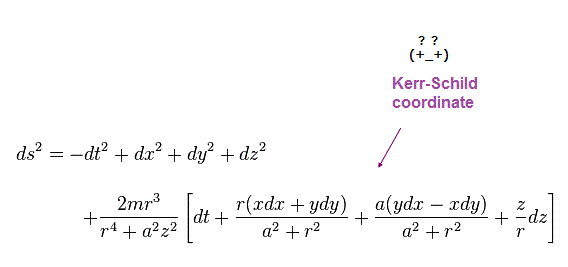In most coordinates, different gravitational pseudotensors give different energy values.  Only under special corrdinate, some of them agree with each other.

See this and this p.12.
This special one is called Kerr-Schild "Cartesian" coordinate ( this p.10 ).

## Derivation of gravitational wave graph.

(Fig.17)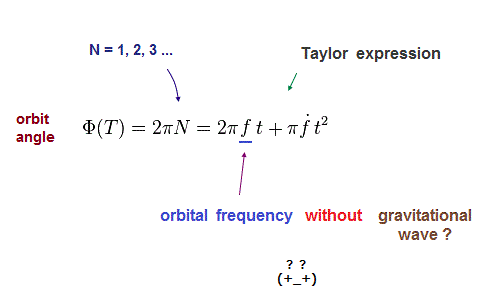Here we show how we can get this graph predicting gravitational wave effect.  After the time "t" passed, the periastron has gone around N times.

So the total orbital angle is 2πN ( N is an integer ).
Fig.17 is Taylor expression to the second-order.

The point is "f" is orbital frequency (= 1/orbital period ) without gravitational wave.  See this and this p.12.

This graph depends on how we decide this frequency value f which doesn't include gravitational wave effect.

But it's impossible to remove only faint gravitational wave effect hidden in large precession !

(Fig.18)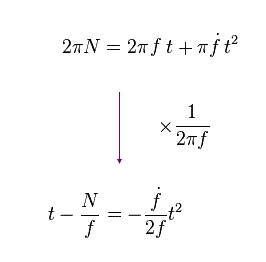From Fig.17, we get Fig.18.
And using orbital period P (= 1/f ), we obtain

(Fig.19)Using measured (= fitted ) parameters of this p.2 in pulsar 1913+16,

(Fig.20)  Pulsar B1913+16 parameters.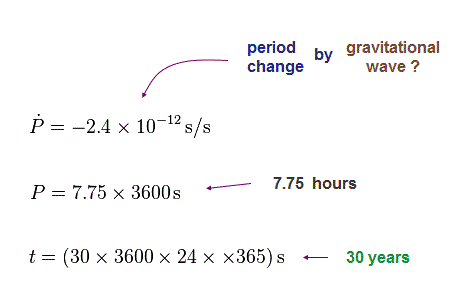We can get the value (= 39 s / 30 years ) of the graph.
This result agrees with this.

(Fig.21)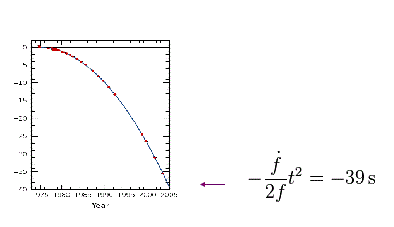The reliability of this prediction of general relativity completely depends on how we determine orbital frequency f ( or period ) excluding gravitational wave.

This gravitational wave effect is too weak to believe.
And it is hidden in other large effects such as precession.